Vector Calculus - 3

# Vector Calculus - 3

Test Description

## 20 Questions MCQ Test Topic-wise Tests & Solved Examples for IIT JAM Mathematics | Vector Calculus - 3

Vector Calculus - 3 for IIT JAM 2023 is part of Topic-wise Tests & Solved Examples for IIT JAM Mathematics preparation. The Vector Calculus - 3 questions and answers have been prepared according to the IIT JAM exam syllabus.The Vector Calculus - 3 MCQs are made for IIT JAM 2023 Exam. Find important definitions, questions, notes, meanings, examples, exercises, MCQs and online tests for Vector Calculus - 3 below.
Solutions of Vector Calculus - 3 questions in English are available as part of our Topic-wise Tests & Solved Examples for IIT JAM Mathematics for IIT JAM & Vector Calculus - 3 solutions in Hindi for Topic-wise Tests & Solved Examples for IIT JAM Mathematics course. Download more important topics, notes, lectures and mock test series for IIT JAM Exam by signing up for free. Attempt Vector Calculus - 3 | 20 questions in 60 minutes | Mock test for IIT JAM preparation | Free important questions MCQ to study Topic-wise Tests & Solved Examples for IIT JAM Mathematics for IIT JAM Exam | Download free PDF with solutions
 1 Crore+ students have signed up on EduRev. Have you?
Vector Calculus - 3 - Question 1

### If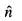is the unit outward drawn normal to any dosed surface S, then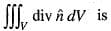Vector Calculus - 3 - Question 2

### Let T (x, y, z) = xy2 + 2z – x2z2 be the temperature at the point (x, y, z). The unit vector in the direction in which the temperature decrease most rapidly at (1, 0, – 1) is

Detailed Solution for Vector Calculus - 3 - Question 2

Let T(x, y), z) = xy2 + 2z – x2z2 be the temperature at a point (x, y, z). Temperature increase most rapidly in the direction of gradient i.e., ∇ T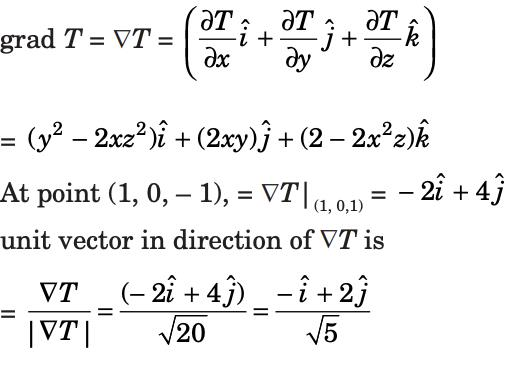So, temperature decreases most rapidly in the direction of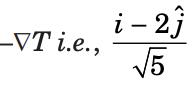.

Vector Calculus - 3 - Question 3

### If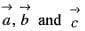are coplanar vectors, then the value of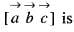Vector Calculus - 3 - Question 4

Let S be the oriented surface x2 + y2 + z2 = 1 with the unit normal n pointing outward. For the vector field F(x, y, z) = xi + yj + zk, the value of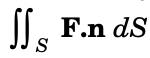is

Detailed Solution for Vector Calculus - 3 - Question 4

By Guass is divergence theorem,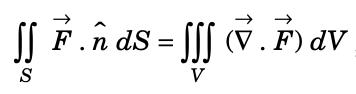,
where V is the volume enclosed by S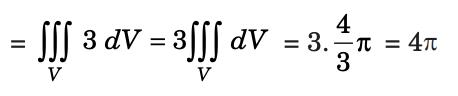since the volume V enclosed by a sphere of unit radius is equal to (4/3)π(13)  = (4/3)π .

Vector Calculus - 3 - Question 5

Let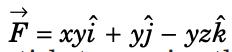denote the force field on a particle traversing the path L from (0, 0, 0) to (1, 1, 1) along the curve of intersection of the cylinder y = x2 and the plane z = x. The work done by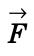is

Detailed Solution for Vector Calculus - 3 - Question 5

Work done by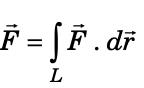,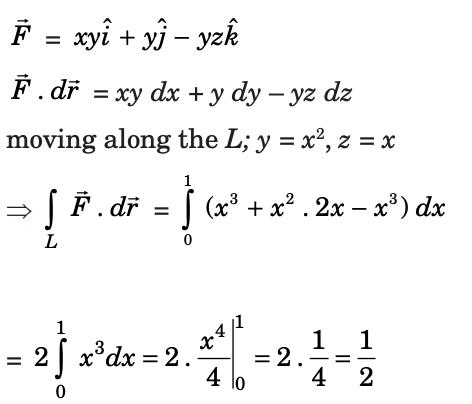Vector Calculus - 3 - Question 6

Stoke’s theorem connects

Vector Calculus - 3 - Question 7

If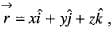then the value of curl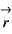will be

Vector Calculus - 3 - Question 8

If φ = 3x2y - y3z2 the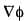at the point (1,-2,-1) is

Detailed Solution for Vector Calculus - 3 - Question 8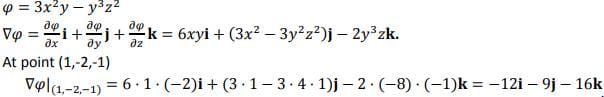Vector Calculus - 3 - Question 9

A vector point function F is said to be solenoidal, if and only if

Vector Calculus - 3 - Question 10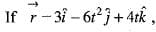then the value of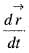will be

Vector Calculus - 3 - Question 11

The work done in moving a particle in the force field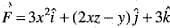along the line joining (0 , 0, 0) to (2 ,1, 3) is

Detailed Solution for Vector Calculus - 3 - Question 11

Explanation : Straight line x = 2t, y = t, z = 3t 0 ≤ t ≤ 1

Work done = ∫F · dr

=  ∫(0 to 1) F·dr/dt * dt

= ∫(0 to 1) F·(dr/dt)dt

= ∫(0 to 1)[3(2t)2i + (2.2t.3t − t)j + 3tk] · [2i + j + 3k]dt

= ∫(0 to 1)[24t2 + 12t2 − t + 9t]dt

= [8t3 + 4t3(1/2t2) + 9/2t2](0 to 1)

= 8 + 4 + 4

= 16

Vector Calculus - 3 - Question 12

The value of curl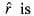Vector Calculus - 3 - Question 13

The mensuration meaning of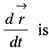Vector Calculus - 3 - Question 14

Green’s theorem in a plane is a special case of

Detailed Solution for Vector Calculus - 3 - Question 14

Green's theorem gives the relationship between a line integral around a simple closed curve, C, in a plane and a double integral over the plane region R bounded by C. It is a special two-dimensional case of the more general Stokes' theorem.

Stokes’ Theorem gives the relationship between a line integral around a simple closed curve, C, in space, and a surface integral over a piecewise, smooth surface.

Green’s theorem in its “curl form”.

where F = P(x,y) i + Q(x,y) j and dr = dx i + dy j

is as follows: (curl form of Green’s Theorem)

c∫ F(x,y) . dr = c∫ F . T ds = r∫ ∫ curl F dA

where curl F is the z-component of curl F = curl F . k

for stoke’s theorem

Vector Calculus - 3 - Question 15

If φ = sin (x + y + 2z) + x2 y3z, then curl grad φ is equal to

Vector Calculus - 3 - Question 16

The value of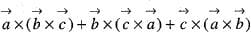is

Detailed Solution for Vector Calculus - 3 - Question 16

Explanation :
a * (b * c) + b * (c * a) + c * (a * b) = 0

L.H.S.
a * (b * c) + b * (c * a) + c * (a * b)
= a * ( a) + b * (b) + c * ( c)
[∵ b * c = a ; c * a = b and a * b = c ]
= a * a + b * b + c * c
= 0 + 0 + 0

[∵ a * a= 0;  b * b = 0 ; c * c= 0]

= 0 + 0 + 0
= 0
= R.H.S.

a * (b * c) + b * (c * a) + c * (a * b) = 0,

Hence proved

Vector Calculus - 3 - Question 17

The volume of the parallelopiped whose edges are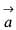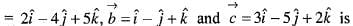Vector Calculus - 3 - Question 18

The value of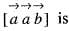Vector Calculus - 3 - Question 19

The value of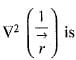Vector Calculus - 3 - Question 20

Consider the vector field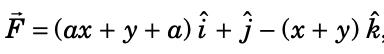,  where a is a constant. If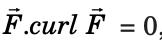, then the value of a is

Detailed Solution for Vector Calculus - 3 - Question 20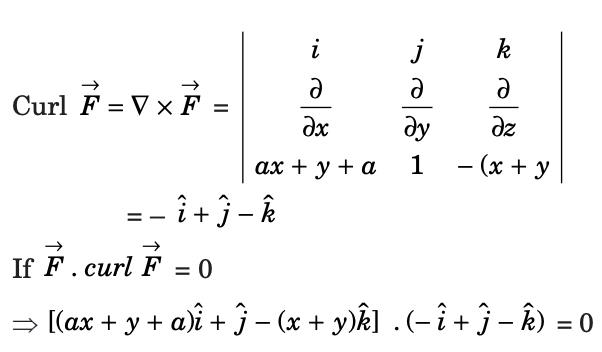⇒ – (ax + y + a) + 1 + x + y = 0
⇒ (– a + 1)x + (– a + 1) = 0
⇒ (– a + 1) (1 + x) = 0
⇒ a = 1 (because x is not constant).

## Topic-wise Tests & Solved Examples for IIT JAM Mathematics

27 docs|150 tests
 Use Code STAYHOME200 and get INR 200 additional OFF Use Coupon Code
Information about Vector Calculus - 3 Page
In this test you can find the Exam questions for Vector Calculus - 3 solved & explained in the simplest way possible. Besides giving Questions and answers for Vector Calculus - 3, EduRev gives you an ample number of Online tests for practice

## Topic-wise Tests & Solved Examples for IIT JAM Mathematics

27 docs|150 tests